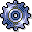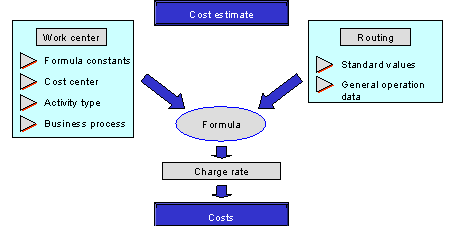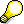RoutingsUse

The routing lists the operations needed to manufacture a product. It specifies the following for each operation:

• The work center at which the operation is carried out
• Which default values are to be used to calculate the dates, capacities, and production costs
• Whether the costs of an operation are taken into account for costing
• The material components needed to carry out an operation

In repetitive manufacturing, you can use rate routings in addition to normal routings and reference operation sets.

Features

For costing, the system selects a routing for the material using the costing variant and its quantity structure determination ID. The planned costs are calculated for each operation.

The system calculates the amount of activity used using the formula in the work center and the default values in the operation of the routing. This activity is valuated using the prices in Cost Center Accounting or Activity-Based Costing to calculate the planned costs for the operation.

The following figure shows the connection between the data in the work center and the data in the routing.Repetitive manufacturing often uses rate routings instead of normal routings. With a routing, the base quantity is normally constant and the times (such as the processing time) are determined through the standard values. With the rate routing, however, it is the other way around. The production quantities are maintained separately for each article, and the time is constant. In a rate routing, therefore, you can specify what quantity of an article is manufactured in a given time using a production rate.

The functions of rate routings and routings are otherwise the same as those of normal routings.